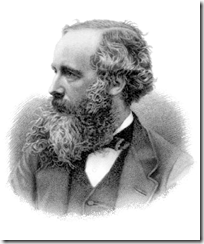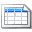# Maxwell's Equations - Introduction

By onJames Clerk Maxwell
James Clerk Maxwell, born in
Edinburgh Scotland 1831 is renowned
for his work on the set of equations
named after him.  Maxwell published
an early form of his equations between
1861 and 1862 (a total of twenty
which were later combined into four
by Oliver Heaviside and Heinrich Hertz).

Maxwell's Equations are a set of fundamental relationships, which govern how electric and magnetic fields interact. The equations explain how these fields are generated and interact with each other, as well as their relationship to charge and current.  They form the backbone of much of modern electrical and telecommunication technology and are often quoted as being the most important equations of all time.

The equations consist of a set of four - Gauss's Electric Field Law, Gauss's Magnetic Field Law, Faraday's Law and the Ampere Maxwell Law.  This note explains the idea behind each of the four equations, what they are trying to accomplish and give the reader a broad overview to the full set of equations.

Follow on notes will deal with each of these equations separately.  These follow on notes, will explain the mathematics (both differential and integral forms) of each law and give example applications.

Follow on notes will deal with each of these equations separately.  These follow on notes, will explain the mathematics (both differential and integral forms) of each law and give example applications.

## Gauss's Electric Field Law

Gauss's Electrical law defines the relation between charge ("Positive" & "Negative") and electric field.  The law was initially formulated by Carl Friedrich Gauss in 1835.

In Gauss's law, the electric field is the electrostatic field.  It shows how the electrostatic field behaves and varies depending on the charge distribution within it.  More formally it relates the electric flux [of the electric field flowing from positive to negative charges] passing through a closed surface to the charge contained within the surface.

The electric field flux passing through a closed surface is proportional to the charged contained within that surface.- integral Form- differential Form

To understand how the equations work and see application examples, please see the following note:

## Gauss's Magnetic Field Law

The magnetic law defines the relationship between magnetic poles and the magnetic field.

It is similar to the electric field law, except that individual magnetic poles ("North" & "South") cannot exist alone (whereas individual charge units can be separated from each other).  That magnetic poles must exist as a pair has a large effect on the behavior of the magnetic flux.

The total magnetic flux passing through a surface is equal to zero.- integral form- differential form

To understand how the equations work and see application examples, please see the following note:

• Maxwell's Equations - Gauss's Magnetic Field Law - yet to be written

It was Michael Faraday, who in 1831 demonstrated through a series of experiments that a conductor enclosing a varying magnetic field will have a current induced.  This in turn, will create an electric field. Transformers and electrical machines all operate on the basis of Faraday's Law.

Another way to view this is that a time varying magnetic field is always associated with a time varying electric field.  And in reverse, with a time varying electric field always associated with a varying magnetic field.

A changing magnetic flux  within a surface induces a electromotive force (EMF) and induced current.  The induced current creates a circulating electric field.- integral form- differential form

To understand how the equations work and see application examples, please see the following note:

• Maxwell's Equations - Faraday's Law - yet to be written

## Ampere Maxwell Law

Discovered in 1826 by  André-Marie Ampère, the law describes the magnetic fields produced by a varying electric current. Ampère initially considered steady electric currents and this was later expanded by Maxwell to included time varying currents.

Changing current or electric flux through a surface produces a changing magnetic field.- integral form- differential form

To understand how the equations work and see application examples, please see the following note:

• Maxwell's Equations - Ampere Maxwell Law - yet to be written

## Maths Review

It is assumed that the reader is familiar with the necessary maths to be able to apply the equations.  If you need to learn or brush up on this, there are good engineering maths textbooks and resources on the Internet.  Having said that, we will give a very quick review of the meaning of the key terms used in the equations.- is the definite integral of function f(x,y,z), taken over a surface S- is the line integral of function f(x,y,z), over a curve C- del (∇) performs derivative operations,and- are the unit vector, vector dot product and vector cross product

### Constants- permittivity of free space (= 8.854187817... F/m)- permeability of free space (= 4π×10−7 ≈ 1.2566370614...×10−6 H/m)

## Summary

This note has introduced the four Maxwell equations:

1. Gauss's Electric Field Law - static electric fields
2. Gauss's Magnetic Field Law - static magnetic fields
3. Faraday's Law - electric fields induced by time varying magnetic fields
4. Ampere Maxwell Law - magnetic fields induced by time varying electric fields

Each of these laws is explained in detail, in associated notes (listed above).   If you have any general comments, please discuss below.  If you have a detailed comment on a particular law, please discuss in the detailed note.

More interesting Notes:Steven McFadyen

Steven has over twenty five years experience working on some of the largest construction projects. He has a deep technical understanding of electrical engineering and is keen to share this knowledge. About the author

#### View 1 Comments (old system)

1.Notes says:
9/4/2013 2:49 PM

Gauss's Electrical law defines the relation between charge ("Positive" & "Negative") and electric field.  The law was initially formulated by Carl Friedrich Gauss in 1835. In Gauss's law, the electric field is the electrostatic field.  The law shows... ...

Comments are closed for this post:
• have a question or need help, please use our Questions Section
• spotted an error or have additional info that you think should be in this post, feel free to Contact Us

Switchboard - Forms of Internal Separation

IEC 61439 'Low-voltage switchgear and controlgear assemblies', specifies standard arrangements of switchboard (call forms of internal separation). The...

For the past few years I have reading eBooks on my HTC touch phone. On and off I have debated buying an eReader and recently purchased a Sony PRS-300 ...

Post Editing Tips

If you at all familiar with programs like office and outlook, then adding and editing posts is pretty straightforward and intuitive.  However, there are...

Fault Calculations - Introduction

Fault calculations are one of the most common types of calculation carried out during the design and analysis of electrical systems. These calculations...

ABB Technical Guides - Motor Operation

ABB has produced a range of technical guides that offer concise explanations of the major technologies and technical issues in low voltage AC drives. ...

Three Phase Current - Simple Calculation

The calculation of current in a three phase system has been brought up on our site feedback and is a discussion I seem to get involved in every now and...

Lighting Design - An Introduction

From the earliest times, humans have found ways to create light. Pre-historic peoples used natural materials (moss, grass, etc.) soaked in animal fat and...

How to Check a Circuit is Dead

If you want to check a circuit is dead (not live), you should always use the three point method. First check a known live circuit, then check the dead...

Closed Doors

"I can live with doubt and uncertainty and not knowing. I think it is much more interesting to live not knowing than to have answers that might be wrong...

Voltage Levels to IEC 60038

The standard aims to consolidate AC and traction voltages within the industry and defines the following bands: band 1 - A.C. systems 100 V to 1...## Have some knowledge to share

If you have some expert knowledge or experience, why not consider sharing this with our community.

By writing an electrical note, you will be educating our users and at the same time promoting your expertise within the engineering community.

To get started and understand our policy, you can read our How to Write an Electrical NoteClick here to view the notes list in a table formatClick here to view the notes indexed by tag